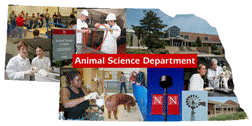## Animal Science Department## Faculty Papers and Publications in Animal Science

#### ORCID IDs

Stephen D. Kachman

#### Date of this Version

4-28-1995

Published in J. Anim. Sci. 1995. 73:2579-2588.

#### Abstract

The nature of the heterogeneity of variance for 200- and 365-d weights by sex, sire breed, and dam breed subclasses was studied. Data consisted of records for weaning f n = 7,829) and yearling (n = 7,367) weights of progeny from 673 and 672 sires, respectively, from 22 breeds that have been evaluated in the Germ Plasm Evaluation Program at the U.S. Meat Animal Research Center, Clay Center, NE. Sires were mated to Hereford and Angus cows. Each trait was analyzed separately. Three studies were undertaken separately to investigate heterogeneity due to the different factors (i.e, sire breed, sex, or dam breed). Only data from seven sire breeds were used to study the factor sire breed, but all data (22 sire breeds) were used to study the factors sex and dam breed. In each study, three sire and dam models with records of animals of the four sex x dam breed combinations considered different traits and with the same model equation, but covariance structures for random effects (sires, dams, and residuals) of increasing generality were fitted. First, (co)variances across subclasses were assumed equal. Second, correlations and fractions of phenotypic variance were assumed equal but phenotypic variance differed by sire breed, sex, or dam breed as appropriate. Third, variances and covariances were different for each subclass of the factor under study. Variance components were estimated by derivative-free REML. Models for each trait and each factor were compared through likelihood ratio tests. For both traits, variances differed ( P < .02) in scale, but not as fractions of phenotypic variance ( P> .l0), by sire breed and sex subclasses. Variances were not different ( P >.l0) by dam breed subclasses, either in scale or as fractions of phenotypic variance. Estimates of correlations among genetic effects on weights of calves from different sex-dam breed subclasses were at least 35. Across all sex, sire breed, and dam breed subclasses, pooled estimates of sire and dam variances as fractions of phenotypic variance were, respectively, .06 and .39 for weaning weight and .l1 and .24 for yearling weight. The conclusion is that the assumption of equal phenotypic variances among sire breeds and between sexes may not be appropriate in genetic evaluations.

COinS